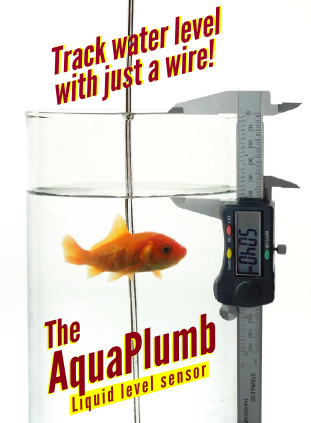Daycounter, Inc. Engineering Services Custom Firmware, Electronics Design, and PCB Layout Home Company Services Products Partners Clients Site Map Contact UsSteinhart-Hart Thermistor CalculatorSee our other Electronics Calculators.

#### Steinhart-Hart Temperature Calculator

Thermistor resistance is  related to temperature in degrees Kelvin by the following formula:

1/T= A + B*ln(R/Rt) + C*ln(R/Rt)2 + D*ln(R/Rt)3

In the standard Steinhart-Hart equation the C parameter is set to zero.  However, some manufacturers use all 4 coefficients.  In the calculator below, you can specify whether to use this term or not, by just setting it to zero.

Subtract 273.15 to convert Kelvin to Celsius.

It's wise to do a quick sanity check by putting in the coefficients and the same value for Rt and R.  If the result isn't 25C then there is a problem with the coefficients.

 Inputs: Coefficient A (K-0) Coefficient B (K-1) Coefficient C (K-2) Coefficient D (K-3) Resistance at ambient temperature Rt (ohms) Measured resistance at T (ohms) Results: Temperature (C)

The coefficients A, B, C are specified over a range of temperatures.  Many times manufactures neglect to put these parameters on a data sheet, and so they must be computed by solving 3 simultaneous equations.  The more narrow the temperature range, the greater the accuracy will be.  Note that the equation is extremely accurate for individual thermistors which have their ABC coefficients individually determined.  However, for a group of thermistors, the resulting temperature can be off due to variances in the lot.  The data sheet should specify the tolerance in %C.

#### Steinhart-Hart Resistance Calculator

We can figure out the resistance at a given temperature using the inverse of the above equation:

R= Rt*exp(A1+B1/T+C1/T2+D1/T3),

where exp is the inverse of ln the natural log.

Note that the values for A1,B1,C1,D1 are different than the coefficients for the equation above!

This calculator will compute a resistance. Note that you can choose whether or not to use the third term, be selecting the check box.

 Inputs: Coefficient A1 (K0) Coefficient B1 (K1) Coefficient C1 (K2) Use this coefficient Coefficient D1 (K3) Resistance at ambient temperature Rt (ohms) T (C) Results: Resistance at T (ohms)

It's wise to do a quick sanity check by putting in the coefficients, and 25C for the temperature, the resultant resistance should be close to Rt.

#### Beta and Alpha Parameters

Most manufacturers will specify alpha and beta, and the R tolerance at the ambient temperature. Beta is temperature dependent, and is specified between two temperature points, and can be used to calculate Temperature between the specified temperatures, with a rated accuracy.  For example, for a beta specified between 25 and 85 it will often be denoted as B25/85. Alpha the temperature coefficient is often denoted as TCR on datasheets.  Alpha is negative for NTC thermistors and positive for PTC thermistors.

Beta is defined as follows:

BT1/T2= 1/(1/T1-1/T2)ln(R1/R2)   (Kelvin)

Thus to calculate R2 you can use this formula:

R2 = R1 / (exp( B*(1/ T1 - 1/ T2) ))

 Inputs: Beta B R1 (ohms) T1 (C) T2 (C) Results: R2 (ohms)

Likewise, we can compute a temperature for a measured resistance:

T2= T1*B/ln(R1/R2)  /  ( B/ln(R1/R2) - T1 )  , Note that T has units of Kelvin.

 Inputs: Beta B R1 (ohms) R2 (ohms) T1 (C) Results: T2 (C)

Alpha can be approximated from Beta as follows:

Alpha= -B/T2 *100

 Inputs: R1 (ohms) R2 (ohms) T1 (C) T2 (C) T for calculating Alpha.  (optional for calculating B) (C) Results: Beta B (K) Aproximate Alpha (TCR) (%/C)

#### Thermal Time Constant

The thermal time constant is a measure of how fast the thermistor can adapt to temperature changes.  If you want to measure quick changes in temperature, then a small time constant becomes important.

Temperature Deviation

Knowing the end accuracy of a family of thermistors can be challenging given the multitudes of parameters on a datasheet.  The first calculation is the total resistance deviation:

Delta(R)= [(1+Delta(RT25))/100)*(1+Delta(B)/100) - 1]*100     (%)

Where Delta represents the tolerance in %.

If we know the Delta(R) at a given temperature, we can use alpha (TCR the temperature coefficient) to figure out the temperature deviation:

Delta(T)= Delta(R) / min(Alpha).

Note that alpha is inversely proportional to temperature.  So we get maximum deviation when alpha is selected at the highest temperature in the range.  From the equation above we can estimate alpha from B at a given temperature.

 Inputs: Beta (K) Delta(Beta): Beta tolerance (%) Delta(RT25): RT25 tolerance (%) Min Alpha (TCR), (%/C) OR Estimate Alpha at Temperature (C) Results: Delta (R) Resistance tolerance (ohms) Delta (T) Temperature tolerance (%/C)

The best way to find electronic parts is to use our free Electronics Component Directory, where a myriad of electronics manufactures are listed.

#### Other Electronics Links

We offer a broad range of electrical engineering calculators and electronics articles and tutorials

Daycounter specializes in contract electronics design.  Do you need some help on your project?  Contact us to get a quote.

Disclaimer:  Daycounter, Inc. doesn't guarantee the result or accuracy of this free calculator.